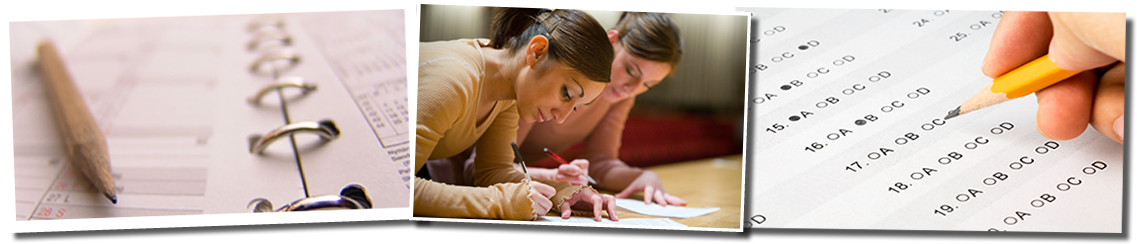# College-Level Math (Math 6)

Evaluation and Testing
1

# College-Level Math (Math 6)

## testing math 3### COLLEGE-LEVEL MATH (MATH 6) TESTING: PLACEMENT INTO ADVANCED MATHEMATICS COURSES

All new students - whether or not they met the University"s college readiness requirement in math are required to take the College-Level Math (Math 6) test. The test score on the Math 6  is used to place students into advanced mathematics or mathematics-related courses and/or meeting your major entry criteria.

Transfer students who have met the math college readiness requirement are generally not scheduled for math placement testing. However, if you wish to register for a math course in your first semester contact the office of Evaluation and Testing to schedule your test.

College-Level Math (Math 6):

• untimed, multiple choice, and computer-based.

• comprised of 20 questions

• external calculators are not allowed. Some, but not all, of the questions contain a  built in pop-up calculator to be use to aid in solving the problem asked. If a question is configured to allow for the use of calculator, the calculator icon will appear in the top right corner of the screen. Depending on the configuration of the question when you click on the calculator icon, you either will see a basic calculator (4-function) or a drop-down menu of multiple calculators that could include basic calculator, square root calculator, or graphing calculator.

• measure the ability to solve problems that involved college-level mathematics concepts

• composed of five types of College-Level Math questions:
1. Algebraic Operations: topics include simplifying rational algebraic expressions, factoring, expanding, polynomials, and manipulating roots and exponents.

2. Solutions of equations and inequalities: topics include the solution of linear and quadratic equations and inequalities, equation systems and other algebraic equations.

3. Coordinate geometry: topics include  plane geometry, the coordinate plane, straight lines, conics, sets of points in the plane, and graph of algebraic functions.

4. Applications and other algebra topics: topics include complex numbers, series and sequences, determinants, permutations and combinations, fractions and word problems.

5. Applications and other algebra topics: topics include polynomials, algebraic, exponential, and logarithmic and trigonometric functions.

You should review the test preparation resources available for the College-Level Math (Math 6) before attending your scheduled testing session.